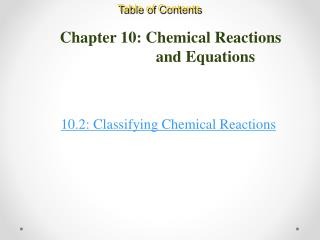# Chapter 10: Chemical Reactions and Equations - PowerPoint PPT PresentationDownload PresentationChapter 10: Chemical Reactions and Equations

Chapter 10: Chemical Reactions and EquationsDownload Presentation## Chapter 10: Chemical Reactions and Equations

- - - - - - - - - - - - - - - - - - - - - - - - - - - E N D - - - - - - - - - - - - - - - - - - - - - - - - - - -
##### Presentation Transcript

1. Table of Contents Chapter 10: Chemical Reactions and Equations 10.2: Classifying Chemical Reactions:

2. Chemical Reactions and Equations: Basic Concepts Recognizing Chemical Reactions • When a substance undergoes a chemical change, it takes part in a chemical reaction. • After it reacts, it no longer has the same chemical identity.

3. Chemical Reactions and Equations: Basic Concepts Recognizing Chemical Reactions • Evidences of chemical reactions: • Change in… • Temperature • Color • Odor • Appearance (sometimes even phase)

4. Chemical Reactions and Equations: Basic Concepts 5 major classes of reactions: 1. Synthesis Reaction A + B → AB CaO(s) + SIO2(l) → CaSiO3(l) 2Na(s) + Cl(g)→ 2NaCl(s)

5. Chemical Reactions and Equations: Basic Concepts 2. Decomposition Reaction AB → A + B MOH(s) → MO(s) + H2O(g) MHCO3(s) → MO(s) + H2O + CO2(g) MCO3(s) → MO(s) + CO2(g)

6. Chemical Reactions and Equations: Basic Concepts 3. Single Displacement/replacement A + BC → B + AC 2Al(s) + Fe2O3(s)→ 2Fe(s) + Al2O3(s) Cl2(aq) + 2KBr(aq) → 2KCl(aq) + Br2(aq)

7. Activity Series • For single displacement to occur: • the “replacing” element MUST be more reactive than the “replaced” element. • See table of reduction potentials (p.288)

8. METALS Lithium Rubidium Potassium Calcium Sodium Magnesium Aluminum Manganese Zinc Iron Nickel Tin Lead Copper Silver Platinum Gold Most active P. 288 HALOGENS Fluorine Chlorine Bromine Iodine Least active

9. Activity Series F2(g) + 2NaBr(aq) → 2NaF(aq) + Br2(l) More active than Br Most active HALOGENS Fluorine Chlorine Bromine Iodine Br2(g) + 2NaF(aq) → NR Less active than F Least active

10. Chemical Reactions and Equations: Basic Concepts 4. Double Displacement/replacement AB + CD → AD + CB PbCl2(s) + BiSO4(aq)→ PbSO4(s) + 2LiCl(aq) BaCl2(aq) + H2SO4(aq) → 2HCl(aq) + BaSO4(s)

11. Chemical Reactions and Equations: Basic Concepts 5. Combustion KEY WORDS: burns explodes combusts CxHyOz + O2→ CO2 + H2O CH4(g) + 2O2(g)→ CO2(g) + 2H2O(g) C6H12O6(s) + 6O2(g) → 6CO2(g) + 6H2O(l) X + O2→ XyOz 2Ba(s) + O2(g) → BaO(s) 4B(s) + 3O2(g) → 2B2O3 (s)

12. Basic Assessment Questions Identify each of the following skeleton equations. Balance each equation if necessary. synthesis decomposition

13. From your homework “Chemical Reactions” Complete and balance the following molecular equations (include phases). Label the type of reaction that will take place • Mg(s) + O2 → • C12H22O11(s)→ • CaCO3(s) + heat →

14. Fin du jour 2 • You should be able to… • Identify types of reactions • Predict products • Look at reactants • What can they form? What is most likely?